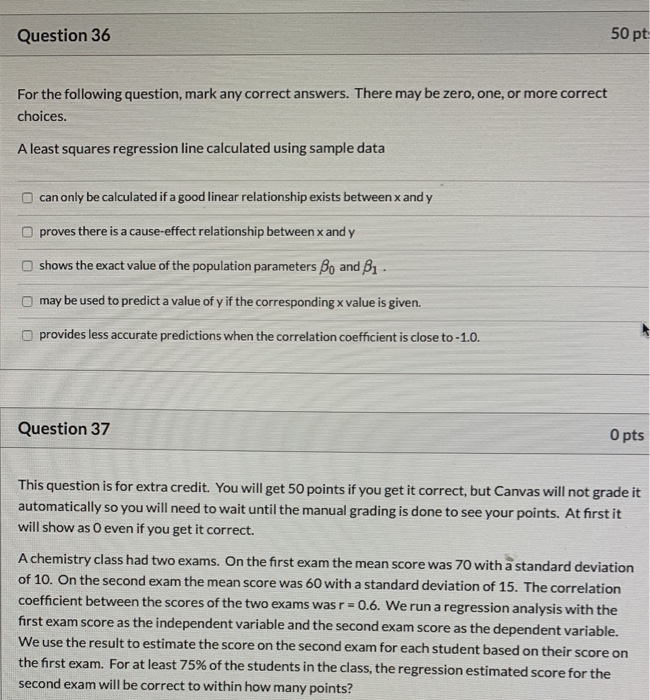Question 36 50 pt For the following question, mark any correct answers. There may be zero, one, or more correct choices. A least-squares regression line calculated using sample data can only be calculated if a good linear relationship exists between x and y proves there is a cause-effect relationship between x and y shows the exact value of the population parameters Bo and Bı. may be used to predict a value of y if the corresponding x value is given. provides less accurate predictions when the correlation coefficient is close to -1.0. Question 37 O pts This question is for extra credit. You will get 50 points if you get it correct, but Canvas will not grade it automatically so you will need to wait until the manual grading is done to see your points.

At first, it will show as O even if you get it correct. A chemistry class had two exams. On the first exam, the mean score was 70 with a standard deviation of 10. On the second exam, the mean score was 60 with a standard deviation of 15. The correlation coefficient between the scores of the two exams was r=0.6. We run a regression analysis with the first exam score as the independent variable and the second exam score as the dependent variable. We use the result to estimate the score on the second exam for each student based on their score on the first exam. For at least 75% of the students in the class, the regression estimated score for the second exam will be correct to within how many points?